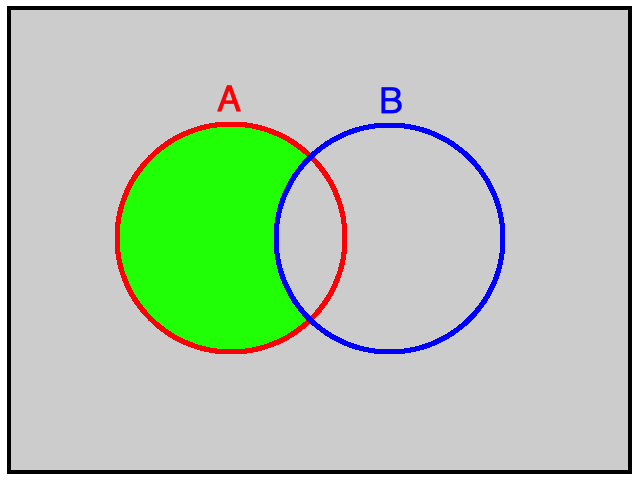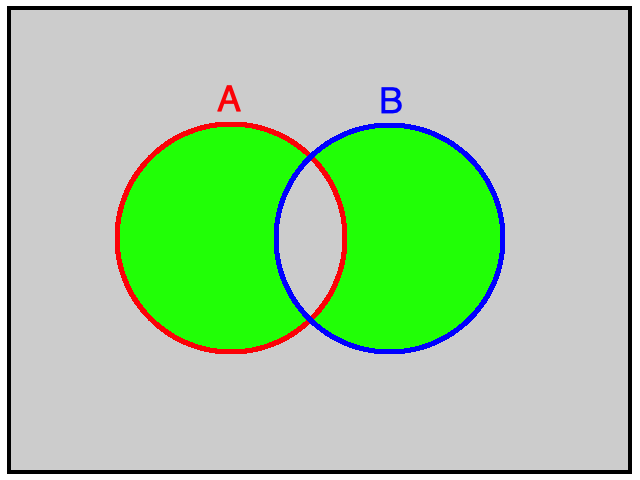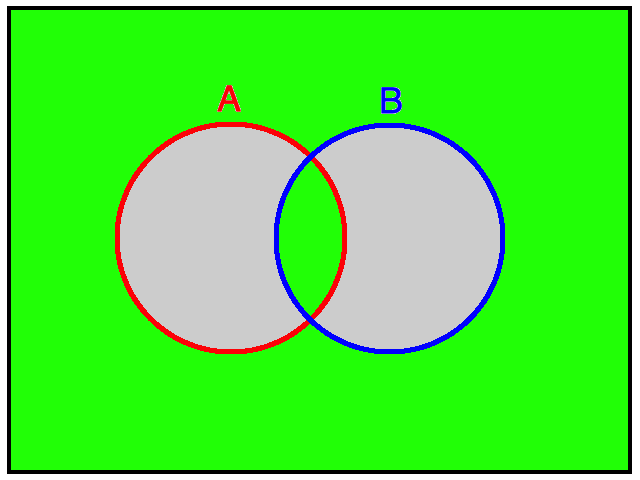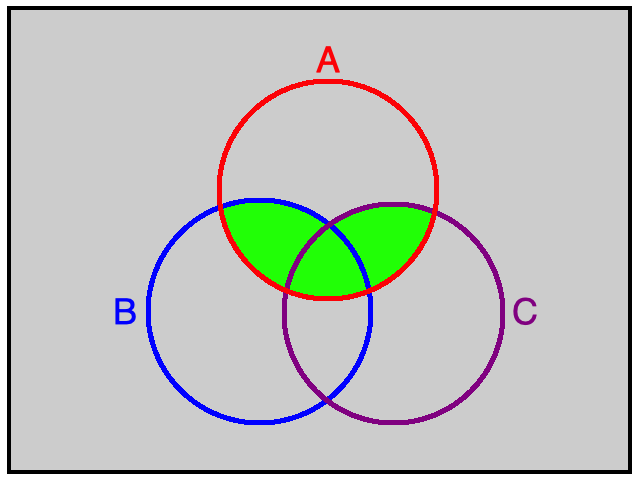## Exercises - Events

1. Venn Diagrams showing sets/events $A$, $B$, and $C$ are shown. Use complements, unions, and intersections to describe each of the sets/events indicated in green.

(a)(b)(c)(d)(e)(f)Answers vary, but the following work:

1. $A \cap \overline{B}$

2. $(A \cap \overline{B}) \cup (\overline{A} \cap B)$

3. $(A \cap B) \cup (\overline{A \cup B})$

4. $A \cap (B \cup C)$

5. $[(A \cap B) \cup (B \cap C) \cup (C \cap A)] \cap \overline{A \cap B \cap C}$

6. $\overline{A \cup B \cup C} \cup (A \cap B \cap C)$

2. Which of the following is not equivalent to either of the other two for given events $A$ and $B$?

1. $P(\overline{A \textrm{ and } B})$
2. $P(\overline{A} \textrm{ or } \overline{B})$
3. $P(\overline{A} \textrm{ and } \overline{B})$

(c) is not the same as the other two.

For the curious, the equivalence of the sets seen in (a) and (b) is known as De Morgan's law.

3. $A \textrm{ xor } B$ (read "A exclusive or B") is the event where exactly one of events $A$ or $B$, but not both, occurs. Develop a rule similar to the addition rule to find $P(A \textrm{ xor } B)$ given the probabilities $P(A)$, $P(B)$, and $P(A \textrm{ and } B)$.

$P(A \textrm{ xor } B) = P(A) + P(B) - 2 P(A \textrm{ and } B)$

4. Develop a rule similar to the addition rule for finding $P(A \textrm{ or } B \textrm{ or } C)$ (Hint: consider a Venn Diagram with 3 sets $A$, $B$, and $C$.).

$$\begin{array}{rcl} P(A \textrm{ or } B \textrm{ or } C) &=& P(A) + P(B) + P(C) \\ && - \,P(A \textrm{ and } B) - P(A \textrm{ and } C) - P(B \textrm{ and } C)\\ && + \,P(A \textrm{ and } B \textrm{ and } C) \end{array}$$